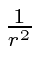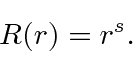## Behavior at the Origin *

The pseudo-potential dominates the behavior of the wavefunction at the originif the potential is less singular than.For small, the equation becomesThe dominant term at the origin will be given by some power ofHigher powers ofare OK, but are not dominant. Plugging this into the equation we getThere are actually two solutions to this equation,and. The first solution,, is well behaved at the origin (regular solution). The second solution,, causes normalization problems at the origin (irregular solution).

Jim Branson 2013-04-22